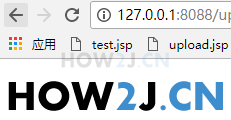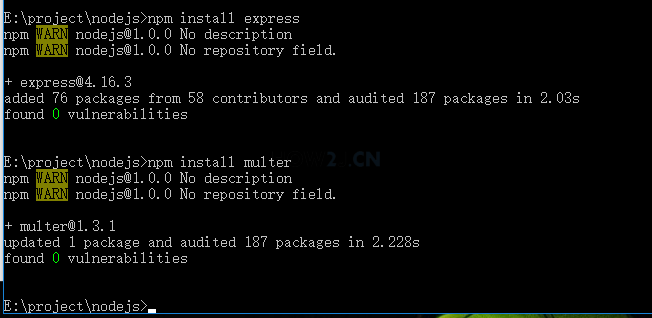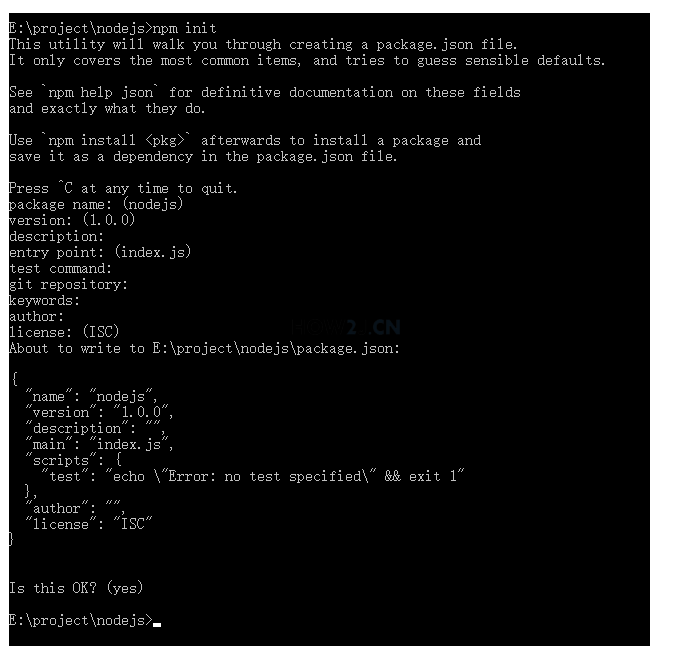node.js系列教材 （十一）- Express 框架 - nodejs 如何 express 访问静态文件 node.js系列教材 （十三）- Express 框架 - 更多

nodejs.rar 933k

## 先运行，看到效果，再学习

node app.js## 准备项目目录

e:\project\nodejs## 安装模块

npm install express

npm install multer## 创建 package.json 目录

npm init<!DOCTYPE html> <meta http-equiv="Content-Type" content="text/html; charset=UTF-8"> <form action="uploadPhoto" method="post" enctype="multipart/form-data"> 上传图片 : <input type="file" name="image" accept="image/*" /> <br> <input type="submit" value="上传"> </form>
```<!DOCTYPE html>

<meta http-equiv="Content-Type" content="text/html; charset=UTF-8">
上传图片 : <input type="file" name="image" accept="image/*" /> <br>
<input type="submit" value="上传">
</form>
```

## app.js

var express = require('express'); var path = require('path') var fs = require("fs"); var multer = require('multer'); var app = express(); //指定静态文件位置 app.use(express.static(path.join(__dirname, 'public'))) //上传之后放在工作目录下的 tmp 目录下。 上传的时候上传控件的name 必须是 image app.use(multer({ dest: path.join(__dirname, 'tmp') }).array('image')); //获取后缀名 function getExtName(fileName){ var index1=fileName.lastIndexOf("."); var index2=fileName.length; var extName=fileName.substring(index1+1,index2); return extName; } app.post('/uploadPhoto', function (req, res) { //获取上传图片的后缀名 var extName = getExtName(req.files.originalname); //随机数 var rundomNumber = Math.ceil(Math.random()*10000000); //以随机数作为文件名 var randomFileName = rundomNumber + "."+extName; //创建图片目录 var imgFolder = __dirname + "/public/img/"; if(!fs.exists(imgFolder)) fs.mkdir(imgFolder); //图片路径 var imgFile = __dirname + "/public/img/" + randomFileName; //上传临时文件的路径 var uploadedTempFilePath = req.files.path; //读取临时文件 fs.readFile( uploadedTempFilePath, function (err, data) { //读取成功之后，复制到图片路径 fs.writeFile(imgFile, data, function (err) { //写成功之后，返回 img元素显示上传之后的图片 res.writeHead(200, {'Content-Type': 'text/html'}); res.end("<img src='img/"+randomFileName+"'/>"); }); }); }) var server = app.listen(8088);

## 运行并测试

node app.jsnode.js系列教材 （十一）- Express 框架 - nodejs 如何 express 访问静态文件 node.js系列教材 （十三）- Express 框架 - 更多

HOW2J公众号，关注后实时获知最新的教程和优惠活动，谢谢。2021-09-28 关于显示乱码问题

2020-12-07 app.js修改好了
 83368126a 关于 前端部分-node.js-上传文件 的提问 代码修改，大家cp一下就行了 ```var express = require('express'); var path = require('path') var fs = require("fs"); var multer = require('multer'); var app = express(); //指定静态文件位置 app.use(express.static(path.join(__dirname, 'public'))) //上传之后放在工作目录下的 tmp 目录下。 上传的时候上传控件的name 必须是 image app.use(multer({ dest: path.join(__dirname, 'tmp') }).array('image')); //获取后缀名 function getExtName(fileName){ var index1=fileName.lastIndexOf("."); var index2=fileName.length; var extName=fileName.substring(index1+1,index2); return extName; } app.post('/uploadPhoto', function (req, res) { //获取上传图片的后缀名 var extName = getExtName(req.files.originalname); //随机数 var rundomNumber = Math.ceil(Math.random()*10000000); //以随机数作为文件名 var randomFileName = rundomNumber + "."+extName; //创建图片目录 var imgFolder = __dirname + "/public/img/"; fs.exists(imgFolder, (exists) => {         if(!exists)             fs.mkdir(imgFolder, (err) => {                 console.log(err);             });     }); //图片路径 var imgFile = __dirname + "/public/img/" + randomFileName; //上传临时文件的路径 var uploadedTempFilePath = req.files.path; //读取临时文件 fs.readFile( uploadedTempFilePath, function (err, data) { //读取成功之后，复制到图片路径 fs.writeFile(imgFile, data, function (err) { //写成功之后，返回 img元素显示上传之后的图片 res.writeHead(200, {'Content-Type': 'text/html'}); res.end(""); }); }); }) var server = app.listen(8088);```

2020-10-06 fs.exists和fs.mkdir的问题
2020-01-31 一直报错，这是怎么回事啊
2019-08-28 为什么这次的图片不是旭旭宝宝了